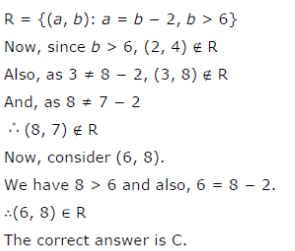# Let R be the relation in the set N given by R = {(a, b): a = b − 2, b > 6}. Choose the correct answer

Let R be the relation in the set N given by R = {(a, b): a = b − 2, b > 6}. Choose the correct answer.
(A) (2, 4) ∈ R
(B) (3, 8) ∈R
(C ) (6, 8) ∈R
(D) (8, 7) ∈ R